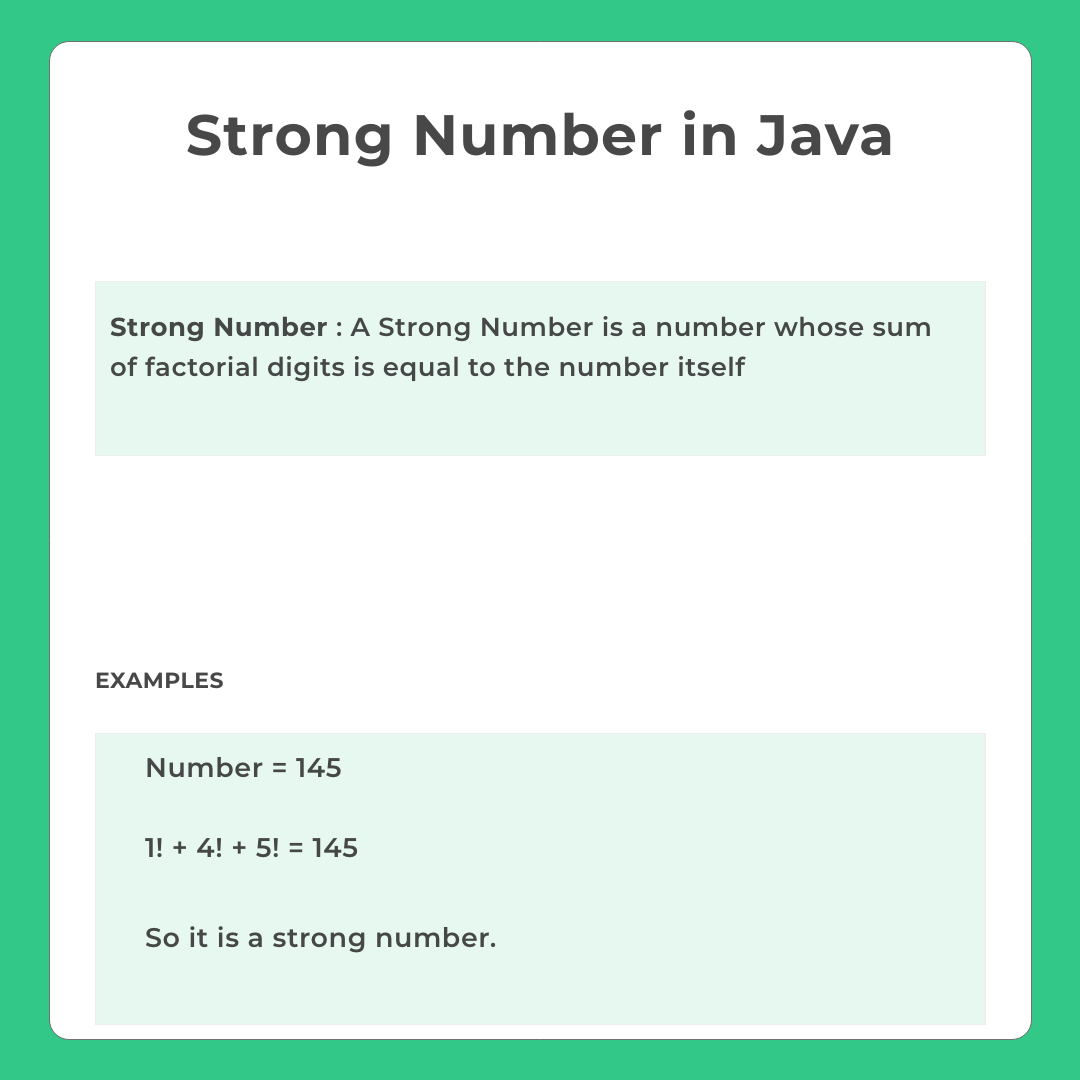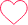# Strong Number Program in Java

## Check Whether or Not the Number is a Strong Number in Java

Given an integer  input the objective is to check whether or not the number is a strong number. Therefore we’ll write a code to Check whether or Not the Number is a Strong Number in Java Language.

```Example
Input : 145
Output : Yes, it's a strong number
```## Check Whether or Not the Given Number is a Strong Number in Java Language

Given an integer input as the number, the objective is to Check Whether or Not the Given Number is a Strong Number in Java Language.

To do so we’ll check if the sum of the factorial of each individual digit of the number is equal to the number itself or not.

For a number to be a Strong Number, the sum of Factorial of each digit of the number must be equal to the number itself. Here are a few method to Check Whether or Not the Given Number is a Strong Number or Not in Java Language,

• Method 1: Using Simple Iteration
• Method 2: Using Recursive Function

We’ll discuss the above mentioned methods in detail in the upcoming sections. Do check out the blue box given below for better understanding of the problem.### Method 1: Using Simple Iteration

In this method we’ll sue the concept of loops and iteration to check whether the number is a strong number or not. To do so we’ll declare a function that takes the number and returns the factorial value. We split the number into individual digits using modulo and divide operators. We then find their factorial using the user defined function. We sum all the factorial values and check if it matches the original number.

### Java Code

Run
```public class Main
{
public static void main (String[]args)
{

int num = 145;

if (detectStrong (num))
System.out.println (num + " is Strong Number");
else
System.out.println (num + " is not a Strong Number");
}

// function to calculate factorial
static int facto (int n)
{
int fact = 1;

for (int i = 1; i <= n; i++)
fact = fact * i;

return fact;
}

static boolean detectStrong (int num)
{

int digit, sum = 0;
int temp = num;
boolean flag = false;

// calculate 1! + 4! + 5!
while (temp != 0)
{
digit = temp % 10;

sum = sum + facto (digit);
temp /= 10;
}

// returns 1 if both equal else 0
if (sum == num)
flag = true;
else
flag = false;

return flag;

}
}```

### Output

`145 is a Strong Number`

### Method 2: Using Recursive Function

In this method we’ll use the concept of recursion to check whether the number is a strong number or not. To do so we’ll first declare a recursive function that takes the number as an argument and returns it’s factorial value. Then we break down the number using the modulo and the divide operator for extracting the digits and shortening the number respectively. We then call the factorial function that we declared before for each number. In the end we sum up all the factorial values and check whether they match the original number.

### Java Code

Run
```public class Main
{
public static void main (String[]args)
{

int num = 145;

if (detectStrong (num))
System.out.println (num + " is Strong Number");
else
System.out.println (num + " is not a Strong Number");
}

// function to calculate factorial
static int facto (int num)
{
if(num == 0)
return 1;

return num * facto(num-1);
}

static boolean detectStrong (int num)
{

int digit, sum = 0;
int temp = num;

// calculate 1! + 4! + 5!
while(temp!=0){
digit = temp % 10;

sum = sum + facto(digit);
temp /= 10;
}

// returns 1 if both equal else 0
return sum == num;

}
}

```

### Output

`145 is a Strong Number`

### Method 3: Smart Dynamic Programming Approach

This method kind of uses dynamic programming to pre-compute factorial values. This helps us as we may not need to re-calculate factorial again and again for new digits

Run
```class Main
{
static int f[] = new int;
// Finding factorial for number 0 to 9
// to precompute factorials without needing them to be calculated again and again
// you can change this 0 to 15 or 0 to 20 for larger values
// in this case change to long
static void preComputer()
{
f = f = 1;
for (int i = 2; i<10; ++i)
f[i] = f[i-1] * i;
}

static boolean checkStrong(int num)
{
int sum = 0;

// traverse individual digits of num
int temp = num;

while (temp > 0)
{
sum += f[temp % 10];
temp = temp / 10;
}
return (sum == num);
}

public static void main (String[] args)
{
// calling preCompute
// this way we do not need to calculate factorial again and again
// we can directly use saved up values like dynamic programming
preComputer();
int val = 145;

if(checkStrong(val))
System.out.println("Its a strong number");
else
System.out.println("Its not a strong number");
}
```

### Output

`Its a strong number`

### Related Banners

Get PrepInsta Prime & get Access to all 200+ courses offered by PrepInsta in One Subscription

## Getting Started

### 6 comments on “Strong Number Program in Java”

•1hk19cs122

public class Main
{
public static void main(String[] args) {
int n = 145,sum=0 ;
int temp = n;
while(temp!=0){
int digit = temp%10;
sum = sum + facto (digit);
temp/=10;

}
if(sum==n){
System.out.println(“It is a Strong Number”);
}else{
System.out.println(“It is not a strong number”);
}
}

static int facto (int n)
{
int fact = 1;

for (int i = 1; i <= n; i++)
fact = fact * i;

return fact;
}
}0
•drakeshkumard

// Using Recursive Function inside a Recursive Function
import java.util.Scanner;
public class StrongNumCheck {
static int factorial(int number){
if(number==0){
return 1;
}
return number * factorial(number – 1);
}
static int sumtotal(int numb){
if(numb==0){
return 0;
}
return factorial (numb%10) + sumtotal (numb/10);
}
public static void main(String[] args) {
System.out.print(“Enter a Number :: “);
Scanner scan=new Scanner(System.in);
int n=scan.nextInt();
if (sumtotal(n) == n){
System.out.println(“Strong NUmber. H O O R A Y ! ! ! !”);
}
else{
System.out.println(“Not a Strong Number.”);
}
}
}0
•Arunima

public class Main
{
public static void main(String[] args) {
int n=40585,temp,r,sum=0;
temp=n;

while(n!=0)
{r=n%10;
int p=1;
for(int j=1;j<=r;j++)
{ p=p*j;
}
sum=sum+p;
n=n/10;
}
if(sum==temp)
System.out.println("s");
else
System.out.println("not");

}
}0
•pamidi

import java.util.*;
class HelloWorld {
int fact(int a)
{
if(a==0 || a==1)
return 1;
else
return a*fact(a-1);
}

public static void main(String arg[])
{
Scanner sc=new Scanner(System.in);
HelloWorld obj=new HelloWorld();
System.out.println(“enter a number :”);
int n=sc.nextInt();
int temp=n;
int sum=0;
while(n>0)
{
int r=n%10;
int res=obj.fact(r);
sum=sum+res;
n=n/10;
}
if(sum==temp)
System.out.println(“strong number”);
else
System.out.println(“not a strong number “);
}
}0
•anurag

package com.company;
import java.util.*;
class Main {
public static void main(String[] args) {
Scanner input = new Scanner(System.in);
System.out.println(“enter the number”);
int n=input.nextInt();
int a=n;
int c=0,d=1,sum=0;
while(a>0)
{
c=a%10;

for(int i = 1;i<=c;i++) {
d = d * i;
}
sum = sum + d;
a=a/10;
c=0;d=1;
}
if(sum==n)
System.out.println("strong");
else
System.out.println("not strong");

}
}1
•Sayan

This code is not working. you have to take another variable, where you sum up all the sum values of factorial. Then you have to check the equality of the variable with the main number.
Here i take the ‘ r ‘ as the final result.
int num = sc.nextInt();
int n = num;
int sum =0 , fac,r=0;
while( n !=0 ) {
fac =1;
int l = n %10 ;
for(int i=l; i >=1; i–) {
fac = fac *i;
sum =+ fac;
}
n = n/10;
r = r + sum;
}
if(r == num) {
System.out.println(“strong number”);
}
else{
System.out.println(“not strong number”);
}0